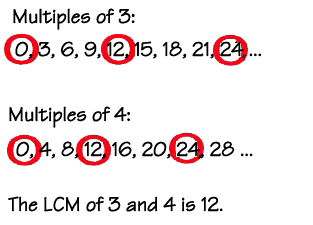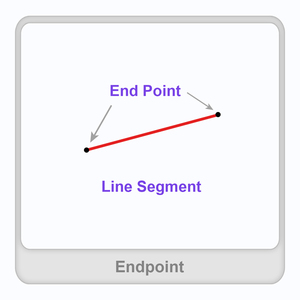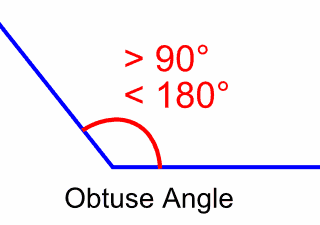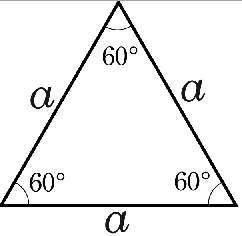Have a Question? Contact me: hforsyth@scholarcharter.org

# Messages

Because homework is meant to be for practice, not for learning a new concept, it will not necessarily be given every night.  The concepts that are being taught in 4th grade Math often require a few days to master.  So, that means that we take more time in class for discussions, hands-on activities, and problem solving. When I feel that the students are confident with the concepts they have been taught, then I will send home the homework for that lesson.  Thank you for your understanding.

## Current Curriculum

In this chapter we will be learning Area and Perimeter.  This includes finding area and perimeter of items in and around your home. They will learn the formulas for doing both of these types of measurements. They will be using many concepts that they have learned before, like division, multiplication, fractions, decimals, and others.  I love when we get into conversions, because it serves as a great review for these concepts as well as learning new ones.  I hope to make this a really fun chapter and your child will be doing several "hands-on" activities.

## Math Vocabulary

Multiplication and Division

Associative Property of Multiplication: The property that states that the grouping of the factors does not change the product.

Commutative Property of Multiplication: The property that states that the order in which two numbers are multiplied does not change the product.

decompose: A way to break down a number into its factors.

dividend: A number that is being divided.

divisor:  The number by which the dividend is being divided.

fact family: A group of related facts using the same numbers.

factor: A number that is multiplied by another number.

Identity Property of Multiplication: The property that states when any number is multiplied by 1, the product is that number (the identity doesn't change).

multiple: The product of a given number and any whole number.

product: The answer of a multiplication problem.

quotient: The answer of a division problem.

repeated subtraction: A strategy that can be used to divide.

Zero property of Multiplication: The property that states that any number multiplied by zero is zero.

Geometry

endpoint: The point at the beginning of a ray or line segment.

line: ct familyA straight set of points that extend in opposite directions without ending.

line segment: A part of a line between two endpoints.  The length of the line segment can be measured.

ray: A part of a line that endpoint and extends in one direction without ending.

point: An exact location represented by a dot.

intersecting: Lines that meet or cross each other.

parallel: Lines that are always the same distance apart.  Parallel lines do not meet.

perpendicular: Lines that meet or cross each other to form right angles.

degree: The unit to measure an angle.

angle:  A figure that is formed by two rays with the same endpoint.

acute angle: An angle with a measure greater than 0 degrees and less than 90 degrees.

obtuse angle: An angle that measures greater than 90 degrees and less than 180 degrees.

right angle: An angle with a measure of 90 degrees.

one-degree angle: An angle that turns through 1/360 of a circle.

protractor: An instrument used to measure angles.

acute triangle: A triangle that has three acute angles.

obtuse triangle: A triangle that has one obtuse angle.

right triangle: A triangle that has one right angle.

quadrilateral: A shape that has 4 sides and 4 angles.

parallelogram: A shape that has 4 sides and opposite sides are equal in length and are parallel.  In addition, opposite angles have the same size.

rectangle: A shape in which opposite sides are equal length and parallel.  It has 4 right angles.

rhombus: A shape in which opposite sides are equal in length and parallel.  It has 4 equal sides.

square: A shape in which opposite sides are equal in length and parallel. It has 4 right angles and 4 equal sides.

trapezoid: A shape that has exactly one pair of parallel sides.

line of symmetry: A line on which a figure can be folded so that its two halves match exactly.

line symmetry: A figure has line symmetry if it can be folded so that the two parts of the figure match or are congruent.

Metric Units of Measure

metric system: the decimal system of measurement.  Includes meter, gram, liter.

millimeter (mm): a metric unit of measuring length. 1/1000 of a meter, .001 of a meter

centimeter (cm) : a metric unit of measuring length.   10 mm = 1 cm, 1/100 of a meter, .01 of a meter

meter (m): a metric unit of measuring length. 100 cm = 1 meter, 1000 mm = 1 m

kilometer (km): a metric unit of measuring length. 1 km = 1000 m

capacity: the amount of liquid a container can hold.  Metric units for measuting capacity = milliliter (mL), and liter (L)

milliliter (mL): a metric unit for measuring capacity.  1000 mL = 1 L, 1/1000 of a L, .001 of a L

liter (L): a metric unit for measuring capacity. 1 L = 1000 mL

mass: the amount of matter that an object has.  Two metric units for measuring mass are gram, and kilogram.

gram (g): a metric unit for measuring mass.  The mass of a penny equals about 1 g

kilogram (kg): a metric unit for measuring mass.  1000 g = 1kg

Customary Units of Measure

Inch or Inches (in.) = about the length of a paper clip

Foot or Feet (ft.) = 12 in.

Yard or Yards (yd.) = 3 ft.

Mile or Miles (mi.) = 5280 ft. or 1760 yd.

Fluid Ounce (fl. oz.): customary unit for measuring capacity

Cup or Cups (c.) = 8 fl. oz.

Pint or pints (pt.) = 16 fl. oz. or 2 c.

Quart or quarts (qt.) = 32 fl. oz., 2 pt., or 4 c.

Gallon or Gallons (gal.) = 128 fl. oz., 4 qt., 8 pt., or 16 c.

Ounce or Ounces (oz.): smallest customary unit of measure for weight

Pound or pounds (lb. or lbs.) = 16 oz.

Ton or Tons (T.) = 2,000 lbs.

# Student Resources

## Multiplication and Division## Multiplication (1-Digit by 2-Digit)## Fractions## Geometry90 degrees = 1/4 turn, 180 degrees = 1/2 turn, 270 degrees = 3/4 turn

360 degrees = 1 full turnWhen measuring an angle,

if the rays point to the right, use the inside measure.If the rays of the angle point left,

use the outside measure.equilateral triangle/acute triangle                         obtuse triangleright triangle